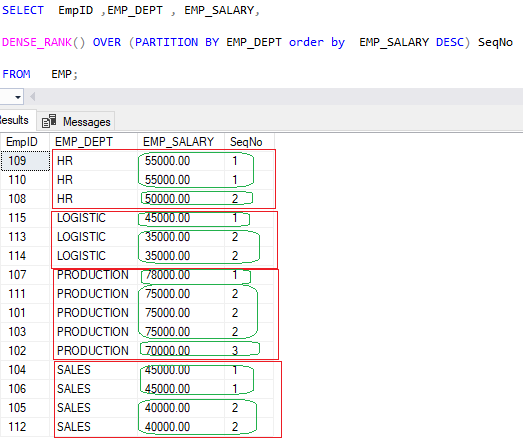Home » SQL Server DENSE_RANK()

# SQL Server DENSE_RANK()The DENSE_RANK() is a ranking function that is used to assign ranks to rows in partitions with no gaps in the ranking values.

If two or more rows in each partition have the same values, they will have the same rank. The next row has the rank increased by one.

It is similar to RANK() function but the only difference is that the DENSE_RANK() function returns consecutive rank values while RANK() function not returns consecutive rank values.

Syntax

```Select DENSE_RANK() OVER (

[PARTITION BY columnnames, ... ]

ORDER BY columnname [ASC | DESC]

) , Col1 , Col2 ,..

from tablename```
PARTITION BY

The PARTITION BY clause divides the result set into partitions.

ORDER BY

The ORDER BY clause defines the logical order of the rows within each partition of the result set.

DENSE_RANK()

DENSE_RANK()  function is operated on the rows of each partition and re-initialized when crossing each partition boundary.

Lets Look at an example of DENSE_RANK() in SQL Server.

First we create a table – EMP, having columns as Department , salary and Employee Name

```Create table EMP
(EMPID INT ,
EMP_DEPT VARCHAR(50) ,
EMP_NAME VARCHAR(100) ,
EMP_SALARY NUMERIC(18,2)
)

Insert into EMP values( 101 ,'PRODUCTION' ,'RAJAT M' ,75000) ,
( 102 ,'PRODUCTION' ,'MUKESH BHATIA' ,70000)
,( 103 ,'PRODUCTION' ,'MUSKAN MEHTA' ,75000)
,( 104 ,'SALES' ,'ROHAN B NARAYAN' ,45000)
,( 105 ,'SALES' ,'SUSHIL DAS' ,40000)
,( 106 ,'SALES' ,'MANISH' ,45000)
,( 107 ,'PRODUCTION' ,'RAJESH SINGH' ,78000)
,( 108 ,'HR' ,'MOHIN KHAN' ,50000)
,( 109 ,'HR' ,'SUSHANT K SINGH' ,55000)
,( 110 ,'HR' ,'LAKSHYA RAWAT' ,55000)
,( 111 ,'PRODUCTION' ,'MANOJ KUMAR' ,75000)
,( 112 ,'SALES' ,'SUJOY M' ,40000)
,( 113 ,'LOGISTIC' ,'VINAY AGARWAL' ,35000)
,( 114 ,'LOGISTIC' ,'MUSTAKIM M' ,35000)
,( 115 ,'LOGISTIC' ,'VIJAY KUMAWAT' ,45000)

```#### Assign a rank with no gaps in ranking values

To assign a rank to employee based on salary with no gaps in ranking values, you can use DENSE_RANK() as given below, you can use DENSE_RANK() as given below.

```SELECT EmpID ,EMP_DEPT , EMP_SALARY,
DENSE_RANK() OVER (order by EMP_SALARY Desc) SeqNo
FROM EMP;
```As you can see, the second ,third and fourth rows share the same rank 2 because they have the same value. The fifth row gets the rank 3 because the DENSE_RANK() function returns consecutive rank .

#### DENSE_RANK() with PARTITION BY

To assign a rank to employee based on salary by partitioning the employee department with no gaps in ranking values, we use DENSE_RANK() as given below.

```SELECT EmpID ,EMP_DEPT , EMP_SALARY,
DENSE_RANK() OVER (Partition By  EMP_DEPT order by EMP_SALARY Desc) SeqNo
FROM EMP;```As we can see, Rank is assigned to employee based on their salaries by partitioning the department and there is no gaps in ranking values department wise.